# Applications of Hydraulics and Pneumatics - MCQs with Answers

## Applications of Hydraulics and Pneumatics – MCQs with Answers

1. In which systems, spool of the servo valve is operated by a torque motor?

a. hydromechanical servo systems
b. electrohydraulic servo systems
c. conventional servo valve
d. all the above

2. What does servo mean in servo valve system?

a. it cannot receive a feedback but the desired output can be obtained
b. it cannot receive a feedback and the desired output cannot be obtained
c. it can receive a feedback and the desired output can be obtained
d. none of the above

ANSWER: c. it can receive a feedback and the desired output can be obtained

3. In conventional valves, which component is used to move the spool?

a. torque motor
b. mechanical servo valve
c. solenoid
d. all the above

4. What is the advantage of DC solenoid coils?

a. DC solenoid coils have high rush in current
b. DC solenoid coils have constant level of current
c. DC solenoid coils have rating of 220 V DC
d. all the above

ANSWER: b. DC solenoid coils have constant level of current

5. Which of the following statements is true for a proportional valve?

a. spool of the proportional valve can travel maximum length
b. digital type of functioning is possible in proportional valve
c. proportional valve requires a separate flow control valve
d. all the above

ANSWER: a. spool of the proportional valve can travel maximum length

6. In which control valve energy consumption reduces as load decreases?

a. conventional direction control valve
b. proportional direction control valve
c. both a and b
d. none of the above

ANSWER: b. proportional direction control valve

7. Which of the following is a characteristic of servo valve?

a. open loop system
b. closed loop system
c. less contamination
d. all the above

8. What is PLC?

a. Process logic control
b. Programmable language converter
c. Programmable logic control
d. Programmable logic converter

9. What causes burning of AC solenoid coil?

a. holding current
b. in rush current
c. current clamps
d. all the above

10. When PLC connections are used instead of electrical connections, the order of operations to be performed can be interchanged by

a. changing hardwired connections
b. changing sequence of program
c. both a and b
d. none of the above

ANSWER: b. changing sequence of program

11. Which of the following is used an output device on a ladder diagram?

a. proximity sensor
b. detent switch
c. relay
d. all the above

12. The output device on a ladder diagram is represented by

a. square
b. circle
c. rectangle
d. semicircle

13. Which of the following circuit shows NOR connection?

a.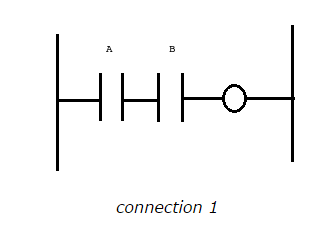b.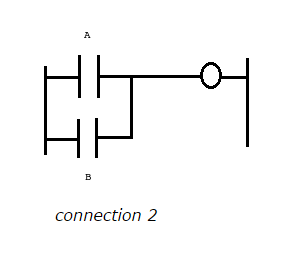c.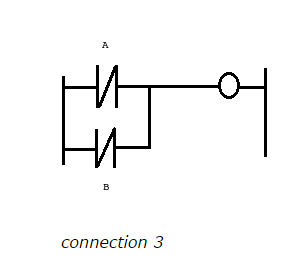d.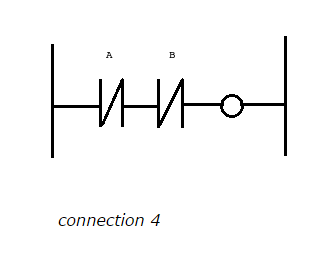14. Write instructions for the NAND connections given below, which has A and B two switches connected in parallel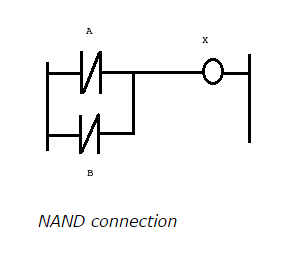a.
steps          0   1   2

components  A   B   X

instruction    LDI ANI OUT

b.
steps          0   1   2

components  A   B   X

instruction    LD AND OUT

c.
steps          0   1   2

components  A   B   X

instruction    LD OR OUT

d.
steps          0   1   2

components  A   B   X

instruction    LDI ORI OUT

15. In mnemonics instructions, what does I in LDI indicate?

a. switch is normally open
b. switch is normally closed
c. it indicates operating of second switch
d. none of the above